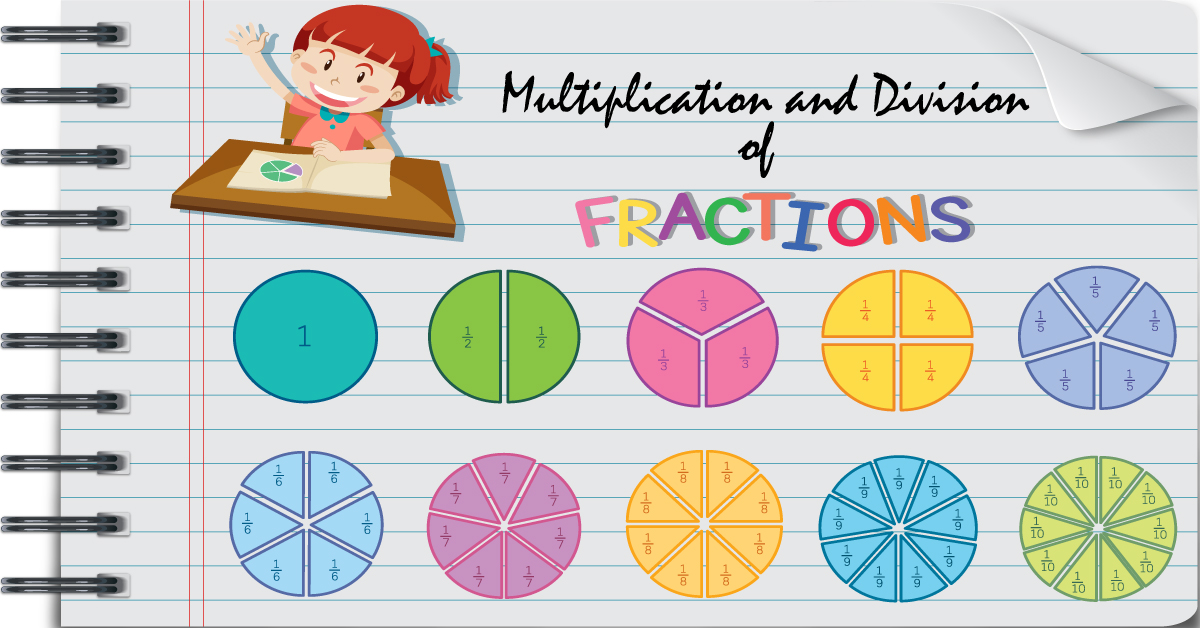# Multiplying and Dividing Fractions

The truth is multiplying and dividing fractions are easier than adding and subtracting fractions. It does not require a lot of steps because it only involves multiplying and simplifying.

When multiplying fractions, you take the numerators and multiply them. You also do the same with the denominators. If needed, the final product is then simplified by dividing the numerator and the denominator by their Greatest Common Factor. Dividing fractions is almost the same as multiplying fractions except that you get the reciprocal of the divisor before proceeding to multiplication and then simplifying the quotient if needed. Simplifying fractions will make computation easy.

But what really happens when you multiply or divide fractions?

## Multiplying Fractions: How Do You Multiply Fractions?

In whole numbers, multiplication can be thought of as repeated addition. For example, $4\times 3$ means adding 3 to itself 4 times:

$4\times 3= 3+3+3+3=12$

Another example is when we multiply a whole number by a fraction.

$3\times \frac{1}{2}= \frac{1}{2}+\frac{1}{2}+\frac{1}{2}=12=\frac{3}{2}$ or $1\frac{1}{2}$

This concept is not always the easier method, especially when we multiply fractions. The thing to remember about multiplying fractions is that you are repeatedly increasing part of a whole. This means, unlike multiplying whole numbers, multiplying fractions results in a product that is even smaller than the factors.

Example:

$\frac{1}{2}\times\frac{1}{3}$ means you are multiplying $\frac{1}{2}$ by less than a whole, in this case, $\frac{1}{3}$

So that if you have $\frac{1}{2}$ of a rectangle, multiplying it by $\frac{1}{3}$ simply means taking a third of that half.

The result is $\frac{1}{6}$ of the whole rectangle.

Another way to look at it is by dividing $\frac{1}{2}$ of the rectangle into 3 equal parts and describing the result in relation to the whole rectangle.

Always remember that if you are multiplying proper fractions, your product will be smaller than the two factors.

## Dividing Fractions: How Do You Divide Fractions?

In whole numbers, for example, $15\div3$ means how many 3 goes into 15. The answer is 5 because there are 5 sets of 3 in 15. The same goes for dividing fractions. You want to know how many parts can go into a given fraction.

Let’s use the same fractions in the multiplication example so you can differentiate the two operations.

$\frac{1}{2}\div\frac{1}{3}=$ This means how many $\frac{1}{3}$ of a whole can you get from $\frac{1}{2}$.

The rectangle is divided in half with a red line. The broken blue lines divide the same rectangle into three to represent thirds. So that by taking half of the rectangle, you get $\frac{1}{3}$ and half of the second$\frac{1}{3}$

$\frac{1}{2}\div\frac{1}{3}=\frac{3}{2}$ or $1\frac{1}{2}$(of the third)

To solve $\frac{1}{2}\div\frac{1}{3}$ without drawing the fractions

Step 1: Get the reciprocal of the divisor

$\frac{1}{3}$ is the divisor and its reciprocal is $\frac{3}{1}$.

Step 2: Change the operation from division to multiplication

$\frac{1}{2}\times\frac{3}{1}$

Step 3: Multiply the fractions.

$\frac{1}{2}\times \frac{3}{1}=\frac{3}{2}$ or $1\frac{1}{2}$

Do not forget to simplify your product by their GCF if needed.

Multiplying and Dividing Fractions are easy especially once you’ve mastered the basic facts. Unlike Addition and Subtraction of Fractions, representing these equations may not be the simplest and the fastest, but sometimes, doing so gives you a better understanding of what actually happens to fractions when they are multiplied or divided.

SummaryArticle Name
Multiplying and Dividing Fractions
Description
This is article will teach you how to solve multiplying and dividing fraction numbers. Follow the easy steps mentioned above and you will master multiplying and dividing fractions in no time.
Author
Publisher Name
Learn ZOE
Publisher Logo